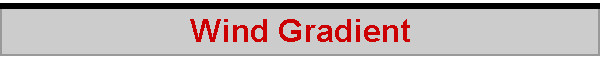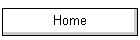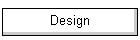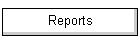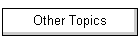Trimming the sails to the wind, as first approximation, is relatively straightforward: they need to develop an angle of attack of about 15 degrees to the oncoming apparent wind (for the beat or the reach). Sailing at an angle of 45 degrees to the true wind, the apparent wind would be at about 30 degrees, and so the jib sheeting angle is about 15 degrees to allow the jib to develop about 15 degrees angle of attack to that apparent wind. This would be fine if the wind was a constant speed up and down the length of the luff, but it isn't. Right down at water level, wind speed is effectively zero. The closer to the water, the slower the wind; this is the wind gradient, caused by friction between the wind and the water surface and its waves. An angle of attack which is correct at deck level is not correct at mast-top level. At deck level, the apparent wind is closer to the bows because the true wind speed is slower. At the mast-top, the apparent wind is closer to the beam because the true wind speed is faster. This is (part of the reason) why the sail must twist, allowing its angle of attack to remain relatively constant to the apparent wind as that apparent wind frees off with increasing height above the water. The interesting question is, by how much does the wind slow down as it approaches the water? What is the wind gradient? Rod Carr very kindly gave me some data he had collected about the wind gradient, and drew my attention to a research article by Ruggles (1970). After analysing Rod's data, I came up with this graph: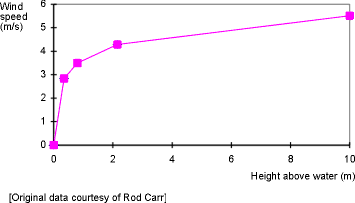The shape of the curve is logarithmic, and uses the formula provided by Ruggles (1970), where the speed of the wind U at a particular height z is given by: U = U*/k LN (z/z0) where k is a constant equal to 0.42, and U* and z0 are two parameters called "friction velocity" and "roughness length". For Carr's data, the curve shown has U*=0.335 and z0=0.01. The value of U*, the friction velocity, somewhat depends upon free wind speed. Carr's data shows an average free wind speed at 10m of about 5.5m/s, and Ruggles (1970) suggests that, on average, U* = 4% of the free wind speed at 10m above the surface. I personally find U* a difficult parameter to interpret, and it seems to me that it is just a "useful" fudge number to make sure that the gradient curve ends up at the free wind height of 10m with the correct speed of the wind for that height. The value of z0, the roughness length, is more interesting, and is an indicator of the roughness of the surface over which the wind gradient is established -- ie how big and rough the waves are. Carr's data suggests z0=0.01 for the average of his observations. Ruggles (1970) suggests that a value of z0=.001 or 0.01 reflects a calm sea and light airs (Beaufort scale 0 or 1), 0.5 modest ripples and gentle breezes (Beaufort 2 or 3), 5.0 modest waves and moderate breezes (Beaufort 4), and 20 substantial waves and fresh breezes (Beaufort 5 or 6). The spreadsheet model (about 28kb) plots a variety of wind gradients using the logarithmic formula, with a variety of roughness lengths and friction velocities. One set of gradients is plotted on the assumption there are no waves; another set on the assumption that waves get uniformly rougher as wind speed increases; and the third set is plotted from Ruggle's (1970) actual data just for interest. Choose which one you like best! If we look at the wind gradient relevant to an IOM -- from deck level to about 1.8m above the water -- we see that the gradient is rather modest at mid-mast, and only becomes significant near deck level. However, near deck level is where most of the sail area is, so we need to take care...When the free wind speed is about 5.5m/s, at the top of the mast the wind speed is around 4.1m/s, and this drops down to 3.5m/s at mid-mast and then down to about 2m/s at boom level (ripples, rather than waves, on the water). If we assume the yacht is on the beat, making 2m/s at an angle of 45 degrees to a true wind of 4.1m/s, the apparent wind angle is about 30.6 degrees at the top of the mast. At mid-mast, where the wind speed is down to 3.5m/s, the apparent wind angle is about 28.9 degrees. Down at boom level, however, the true wind speed of 2m/s gives an apparent wind angle of 22.5 degrees. Ignoring all other factors, we therefore need about 8 degrees of twist (30.6 - 22.5 = 8.1) to the sail, and should sheet the boom in to about 7.5 degrees. Ruggles, KW (1970). The vertical mean wind profile over the ocean for light to moderate winds. J Applied Meteorology, 9, 389-395. 2018-06-22